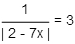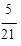# IFoA_CAA_M0 Certified Actuarial Analyst CAA Exam Practice Test

Page:    1 / 14
Total 64 questions

### Question 1

A discrete random variable can only take the values 2,3,4 or 5. The probabilities associated with some of the outcomes are: P(X=2) = 0.2, P(X=3) = 0.3, P(X=5) = 0.1.

For a randomly drawn value of X, calculate P(X>3).

### Question 2

One of the two solutions to the equation is .Determine the second solution.### Question 3

The particular solution of the second-order difference equation

yn+2 - 6yn+1 + 8yn = 0 n 2

subject to the initial conditions y0 = 3 and y1 = 2may be written in the form

yn = A(2n) + B(4n) n 0.

Determine the values of (A, B).

### Question 4

Determine the coefficient of x3in the binomial expansion of (2 + x)5.